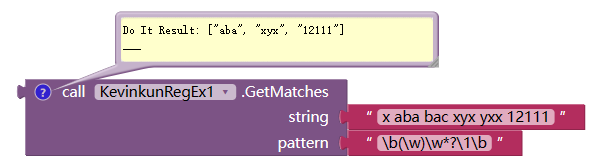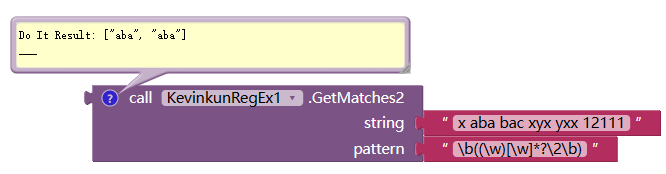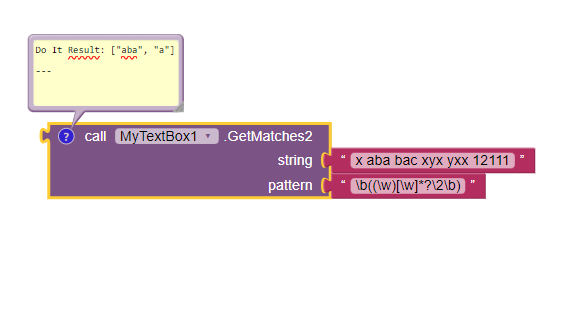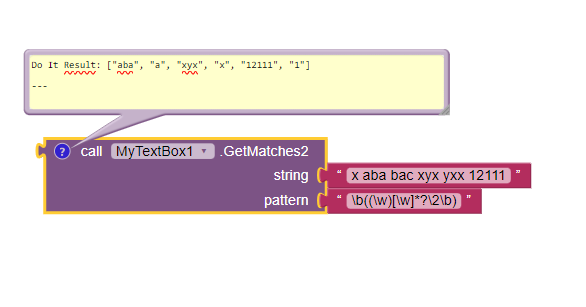# Regular Expression

My text has 150 pages and it has , or ; or ! or .....

Can you give another example of input and output of what you want?

i.e. i have aaa bbb ccc ddd and i want to get aa bb cc

Another example:
I would like in a text, to search for all the words followed by the character "; ".
More generally, I would like to use regex in App inventor because I'm used to doing it in other languages.

Very interesting method, I had not thought of it. THANKS.
Nevertheless, the complexity of the implementation removes all the charm of regex.

How to recover capture groups with Kevinkun.
regex = "\b((\w)[\w]*?\2\b)"
text = "x abaa, bac; 1211."

KevinkunRegex extension can capture groups, but you need to figue out how to write the right regular expression.You do not retrieve capture groups but only complete matches.
If I need a smaller group, for example (\w) I don't know how to get it.

here is the source code of GetMatches

``````	public List<String> GetMatches(String string, String pattern) {
List<String> ls = new ArrayList<String>();
Pattern p = Pattern.compile(pattern);
Matcher m = p.matcher(string);
while (m.find()) {
}
return ls;
}
``````

I have no idea how to change it to meet your need.

I prepare the specifications of only two functions:
Regexp(text,reg,flag,start)
RegexpReplace(text,reg,replace)
and I study java regex, in particular m.group()
then I come back to you.

import java.util.regex.Matcher;
import java.util.regex.Pattern;

public class RegexGroup {
public static void main(String[] args) {
Pattern pattern = Pattern.compile("i(s)");
String input = "My name is Khan and m not a terrerist.";
Matcher m = pattern.matcher(input);
m.find();
String grp0 = m.group(0);
String grp1 = m.group(1);
System.out.println("Group 0 " + grp0);
System.out.println("Group 1 " + grp1);
System.out.println(input);
}
}

output:
Group 0 is ----------> full match of occurrence 1
Group 1 s ----------> captured group number 1 of occurrence 1

in fact I alread tried with this:

``````	@SimpleFunction(description = "获取符合规则表达式的片段，返回列表")
public List<String> GetMatches2(String string, String pattern) {
List<String> ls = new ArrayList<String>();
Pattern p = Pattern.compile(pattern);
Matcher m = p.matcher(string);
m.find();
for (int i = 0; i < m.groupCount(); i++) {

}

return ls;
}
``````

and I got this:Try changing:

``````for (int i = 1; i <= m.groupCount(); i++) {
``````

So that it would show group 1 and 2 instead of 0 and 1.

I tested in a compiled extension:``````@SimpleFunction(description = "获取符合规则表达式的片段，返回列表")
public List<String> GetMatches2(String string, String pattern) {
List<String> ls = new ArrayList<String>();
Pattern p = Pattern.compile(pattern);
Matcher m = p.matcher(string);
while (m.find()) {
for (int i = 1; i <= m.groupCount(); i++) {
}
}
return ls;
}
``````Project:

Only one bloc !
Change "error in pattern" to "error in pattern or start"

EX1
Regexp("Aujourd'hui c'est Dimanche", "\bDi")
---> 1
Regexp("Aujourd'hui c'est dimanche", "\bDi")
---> 0

EX2
Regexp("Dimanche Lundi Mardi Mercredi", "\b\w+di\b", 1)
---> [1, [15, "Lundi"]]

EX3
Regexp("Dimanche Lundi Mardi Mercredi", "\b(\w+)(di)\b", 1)
---> [1, [15, "Lundi", "Lun", "di"]]

EX4
str="Dimanche Lundi Mardi Mercredi"
list = Regexp(str, "\b(\w+)(di)\b", 1)
while list = 1
#traiter list
list = Regexp(str, "\b(\w+)(di)\b", 1, list)

--->[1, [15, "Lundi", "Lun", "di"]]
--->[1, [21, "Mardi", "Mar", "di"]]
--->[1, [30, "Mercredi", "Mercre", "di"]]
--->[-1]

EX5
str="Dimanche Lundi Mardi Mercredi"
list = Regexp(str, "\b(\w+)(di)\b", 2)

--->[1, ["Lundi", "Lun", "di"], ["Mardi", "Mar", "di"], ["Mercredi", "Mercre", "di"]]

I added 3 blocks, the block name and above image explains what they can do.

Kevin, it's not a personal need to be able to access captured groups but the need of any serious regexp user.
I will test your new version.
Cordially

It still lacks ReplaceAll which supports groups.

EX1
We want to replace all vowels with @
str=""Where have all the flowers gone?"
str=RegexpReplace(str, "[aeiou]", "@")
---> "Wh@r@ h@v@ @ll th@ fl@w@rs g@n@?"

EX2
We want to change the format of a date
str="date: 14:27 28/03/2023"
str=RegexpReplace(str, "(\d{2})/(\d{2})/(\d{4})", "\$2.\$1.\$3")
---> "date: 14:27 03.28.2023"

\$0 is full match: "28/03/2023"
\$1 is captured group number 1: "28"
\$2 is captured group number 2: "03"
\$3 is captured group number 3: "2023"
The "/" is not captured, is remplaced by "."

Cordially